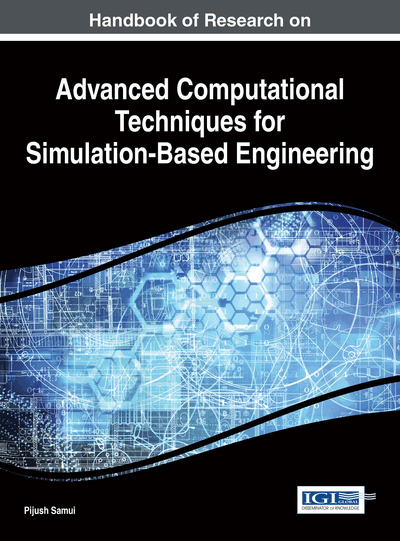# Adaptive Fuzzy Modeling using Orthonormal Basis Functions for Network Traffic Flow Control

Flávio Henrique Teles Vieira (Federal University of Goiás, Brazil), Flávio Geraldo Coelho Rocha (Federal University of Goiás, Brazil) and Álisson Assis Cardoso (Federal University of Goiás, Brazil)
DOI: 10.4018/978-1-4666-9479-8.ch011

## Abstract

In this chapter, we present some Fuzzy training algorithms, such as the Fuzzy LMS (Least Mean Squares) and Fuzzy RLS (Recursive Least Squares) predictors. We use concepts of multifractal analysis to present and validate a Fuzzy LMS predictor based on the autocorrelation function of a multifractal model. We evaluate the efficiency of these algorithms when applied to bandwidth allocation tasks. We also present adaptive predictive OBF (Orthonormal Basis Functions)-Fuzzy models. To this end, we model traffic traces using OBF functions obtained through multifractal analysis. Further, we insert these functions into OBF-Fuzzy models trained with the adaptive training algorithms. Updating the Fuzzy model parameters, we predict future values of real traffic traces. We also present a comparison of prediction performance of different adaptive Fuzzy algorithms including OBF-Fuzzy models. Finally, we verify the performance of the OBF-Fuzzy algorithms in modeling the buffer queueing in a communication network and controlling traffic flow rates.
Chapter Preview
Top

## Introduction

Among the computational models presented in the literature, Fuzzy models deserve attention. Many studies show that they have advantages over linear models in describing the nonlinear time-varying characteristics of unknown real processes in numerous industrial applications and academic researches (Chen, Peng, & Wang, 2000) (Kamik & Kamur, 2005). In fact, the Fuzzy modeling is capable of representing a nonlinear complex system through the combination of linear local models (Takagi & Sugeno, 1985). Specifically, the Fuzzy model described in (Kim, Ji, Park, & Park, 1997) is able to describe an unknown system using a small number of Fuzzy rules such as the model proposed by Takagi and Sugeno (Takagi & Sugeno, 1985) while being easy to implement as the model of Sugeno and Yasukawa (Sugeno & Yasukawa, 1993). However, these studies do not present adaptive training algorithms for the Fuzzy models. Thus, the modeling process of time series requires the processing of all their samples.

Adaptive algorithms are more suitable for real-time applications due to faster information processing. Some areas of computer networks, such as network congestion control, can be benefited by adaptive algorithms implementations. In fact, several congestion control schemes for computer networks have been proposed in the literature (Lee C. C., 1990) (Durresi, Sridharan, & Jain, 2006) (Kamik & Kamur, 2005) (Nejad, Yaghmaee, & Tabatabaee, 2006) (Wang, Liu, Sohraby, & Hou, 2007). Among the proposed congestion control using Fuzzy logic, some are not adaptive such as in Hu and Petr (Hu & Petr, 2000) and others are based on specific protocols or network technologies (Chen, Yang, Lee, & Lee, 2003) (Nejad, Yaghmaee, & Tabatabaee, 2006). In the first case, many of the schemes are not sufficiently accurate in predicting the traffic variation behavior generated by real-time applications due to their failure to adapt the model parameters (Pitsillides & Lembert, 1997). For example, in Hu and Petr (Hu & Petr, 2000), the authors propose a Fuzzy based algorithm for predictive control of traffic flow rates. However, in this approach the membership functions that characterize the Fuzzy predictor inputs are fixed, i.e., such functions are not updated as new inputs are available. In the second case, we can cite control schemes that are exclusives to the protocols of ATM networks (Asynchronous Transfer Mode) (Chen, Yang, Lee, & Lee, 2003) (Kharaajoo, 2004) or based on the congestion control mechanisms of TCP/IP (Transmission Control Protocol/Internet Protocol), as in (Basar & Srikant, 2005) (Wang, Liu, Sohraby, & Hou, 2007) (Wang, Yang, Qiao, & Wang, 2006). Among the congestion control proposals that do not depend on specific network mechanisms, we highlight the scheme presented by (Adas, 1998) (Molnar & Terdik, 2001), which uses the LMS (Least Mean Squares) adaptive algorithm (Marquardt, 1963) for network traffic prediction and control of traffic flow rates. In (Chong, Li, & Ghosh, 1995) (Toreyin, Trocan, & Cetin, 2006), another work in this direction, the authors use the RLS (Recursive Least Squares) algorithm that has faster convergence, to predict the necessary traffic rates (Dang, Molnar, & Maricza, 2003).

## Complete Chapter List

Search this Book:
Reset